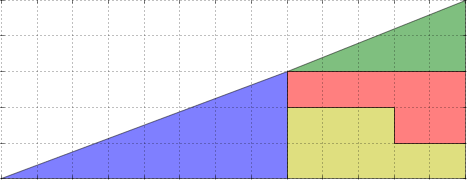# A Simple Animation: The Magic Triangle

I've been spending a lot of time lately playing with animations in IPython notebook. Here's my latest one - a slightly puzzling rearrangement of shapes into two different triangles:Where does the extra block go? Look close and you might be able to figure it out.

I created the animation using just a few lines of matplotlib. Here we'll display it using the Javascript Animation widget that I wrote:

In :
%pylab inline

Welcome to pylab, a matplotlib-based Python environment [backend: module://IPython.kernel.zmq.pylab.backend_inline].

In :
# JS Animation import is available at http://github.com/jakevdp/JSAnimation
from JSAnimation.IPython_display import display_animation
from matplotlib import animation

# Set up the axes, making sure the axis ratio is equal
fig = plt.figure(figsize=(6.5, 2.5))
ax = fig.add_axes([0, 0, 1, 1], xlim=(-0.02, 13.02), ylim=(-0.02, 5.02),
xticks=range(14), yticks=range(6), aspect='equal', frameon=False)
ax.grid(True)

# Define the shapes of the polygons
P1 = np.array([[0, 0], [5, 0], [5, 2], [0, 0]])
P2 = np.array([[0, 0], [8, 0], [8, 3], [0, 0]])
P3 = np.array([[0, 0], [5, 0], [5, 1], [3, 1], [3, 2], [0, 2], [0, 0]])
P4 = np.array([[0, 1], [3, 1], [3, 0], [5, 0], [5, 2], [0, 2], [0, 1]])

# Draw the empty polygons for the animation
kwds = dict(ec='k', alpha=0.5)
patches = [ax.add_patch(plt.Polygon(0 * P1, fc='g', **kwds)),

# This function moves the polygons as a function of the frame i
Nframes = 30
def animate(nframe):
f = nframe / (Nframes - 1.0)
patches.set_xy(P1 + (8 - 8 * f, 3 - 3 * f + 0.5 * np.sin(f * np.pi)))
patches.set_xy(P2 + (5 * f, 2 * f - 0.5 * np.sin(f * np.pi)))
patches.set_xy(P3 + (8 - 3 * f, 0))
patches.set_xy(P4 + (8, 1 - f))
return patches

anim = animation.FuncAnimation(fig, animate, frames=Nframes, interval=50)
display_animation(anim, default_mode='once')

Out:

Once Loop Reflect

For a brief tutorial on creating matplotlib animations, see my previous post on the subject.

By the way, the animated gif at the head of this post was created using the following script. It's similar to above, except we add some extra frames to create the patrol-loop and the pause at each end.

Note that saving the animation as an animated gif requires both matplotlib version 1.3+ and the imagemagick command.

In :
def animate_as_gif(nframe):
if nframe >= Nframes and nframe < Nframes + 15:
nframe = Nframes - 1
elif nframe >= Nframes + 15 and nframe < 2 * Nframes + 15:
nframe = 2 * Nframes + 14 - nframe
elif nframe >= 2 * Nframes + 15:
nframe = 0
return animate(nframe)

anim = animation.FuncAnimation(fig, animate_as_gif,
frames=2 * Nframes + 30, interval=50)
anim.save('MagicTriangle.gif', writer='imagemagick')


Enjoy!

This post was written in an IPython notebook, which can be downloaded here, or viewed statically here.